# C Operators Assignment Mathematical Relational An operator is a symbol that instructs C to perform some operation, or action, on one or more operands. An operand is something that an operator acts on. In C, all operands are expressions.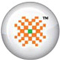PRO DATA DOCTOR it | es | pt | fr | de | jp | kr | cn | ru | nl | gr

Operators

An operator is a symbol that instructs C to perform some operation, or action, on one or more operands. An operand is something that an operator acts on. In C, all operands are expressions. C operators are of following four categories:

• The assignment operator
• Mathematical operators
• Relational operators
• Logical operators

Assignment Operator

The assignment operator is the equal sign (=). The use of equal sign in programming is different from its use in regular mathematical algebraic relations. If you write

x = y;

In a C program, it does not mean "x is equal to y." Instead, it means "assign the value of y to x." In a C assignment statement, the right side can be any expression, and the left side must be a variable name. Thus, the form is as follows:

variable = expression;

During the execution, expression is evaluated, and the resulting value is assigned to variable.

Mathematical Operators

C's mathematical operators perform mathematical operations such as addition and subtraction. C has two unary mathematical operators and five binary mathematical operators. The unary mathematical operators are so named because they take a single operand. C has two unary mathematical operators.

The increment and decrement operators can be used only with variables, not with constants. The operation performed is to add one to or subtract one from the operand. In other words, the statements ++x; and --y; are the equivalents of these statements:

x = x + 1;
y = y - 1;

binary mathematical operators take two operands. The first four binary operators, which include the common mathematical operations found on a calculator (+, -, *, /), are familiar to you. The fifth operator Modulus returns the remainder when the first operand is divided by the second operand. For example, 11 modulus 4 equals 3 (11 is divided by 4, two times and 3 left over).

Relational Operators

C's relational operators are used to compare expressions. An expression containing a relational operator evaluates to either true (1) or false (0). C has six relational operators.

Sample Chapters from book DATA RECOVERY WITH AND WITHOUT PROGRAMMING by Author Tarun Tyagi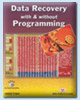Publishers of the Book Number of Pages ISBN Price of the Book BPB Publications, New Delhi, India 540 81-7656-922-4 \$69.00 (Including Shipping Charges, Cost of Book and Other expenses, Free Source Code CD included with the Book)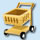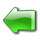page 1 | 2 | 3 | 4 | 5 | 6 | 7 | 8 | 9 | 10 | 11 | 12 | 13 | 14 | 15 | 16 | 17 | 18 | 19 | 20

 page 21 | 22 | 23 | 24 | 25 | 26 | 27 | 28 | 29 | 30 | 31 | 32 | 33 | 34 | 35 | 36 | 37

 page 38 | 39 | 40 | 41 | 42 | 43 | 44 | 45 | 46 | 47 | 48 | 49 | 50 | 51 | 52 | 53 | 54

 page 55 | 56 | 57 | 58 | 59 | 60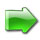© Copyright 2002-2005 DataDoctor.Biz
 Website Data Recovery | recuperación de Datos | Récupération de données | Datenrettung | Recupero dati | データ復旧 | 데이터 복구 | 数据恢复 | Восстановление данных | De terugwinning van gegevens | Ανάκτηση δεδομένων Sitemap Site map1 2 3 4 | Spanish1 2 3 | French1 2 3 | German1 2 3 | Italian1 2 3 | Portuguese1 2 3 | Japanese1 2 3 | Korean1 2 3 | Chinese1 2 3 | Russian1 2 3 | Dutch1 2 3 | Greek1 2 3 Data Recovery Book English | Spanish | French | German | Italian | Portuguese | Japanese | Korean | Chinese | Russian | Dutch | Greek